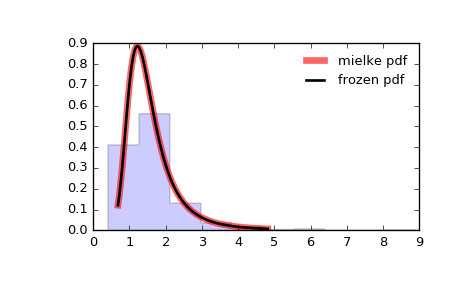# scipy.stats.mielke¶

scipy.stats.mielke = <scipy.stats._continuous_distns.mielke_gen object at 0x2aba950b8b10>[source]

A Mielke’s Beta-Kappa continuous random variable.

As an instance of the rv_continuous class, mielke object inherits from it a collection of generic methods (see below for the full list), and completes them with details specific for this particular distribution.

Notes

The probability density function for mielke is:

mielke.pdf(x, k, s) = k * x**(k-1) / (1+x**s)**(1+k/s)


for x > 0.

mielke takes k and s as shape parameters.

The probability density above is defined in the “standardized” form. To shift and/or scale the distribution use the loc and scale parameters. Specifically, mielke.pdf(x, k, s, loc, scale) is identically equivalent to mielke.pdf(y, k, s) / scale with y = (x - loc) / scale.

Examples

>>> from scipy.stats import mielke
>>> import matplotlib.pyplot as plt
>>> fig, ax = plt.subplots(1, 1)


Calculate a few first moments:

>>> k, s = 10.4, 3.6
>>> mean, var, skew, kurt = mielke.stats(k, s, moments='mvsk')


Display the probability density function (pdf):

>>> x = np.linspace(mielke.ppf(0.01, k, s),
...                 mielke.ppf(0.99, k, s), 100)
>>> ax.plot(x, mielke.pdf(x, k, s),
...        'r-', lw=5, alpha=0.6, label='mielke pdf')


Alternatively, the distribution object can be called (as a function) to fix the shape, location and scale parameters. This returns a “frozen” RV object holding the given parameters fixed.

Freeze the distribution and display the frozen pdf:

>>> rv = mielke(k, s)
>>> ax.plot(x, rv.pdf(x), 'k-', lw=2, label='frozen pdf')


Check accuracy of cdf and ppf:

>>> vals = mielke.ppf([0.001, 0.5, 0.999], k, s)
>>> np.allclose([0.001, 0.5, 0.999], mielke.cdf(vals, k, s))
True


Generate random numbers:

>>> r = mielke.rvs(k, s, size=1000)


And compare the histogram:

>>> ax.hist(r, normed=True, histtype='stepfilled', alpha=0.2)
>>> ax.legend(loc='best', frameon=False)
>>> plt.show()Methods

 rvs(k, s, loc=0, scale=1, size=1, random_state=None) Random variates. pdf(x, k, s, loc=0, scale=1) Probability density function. logpdf(x, k, s, loc=0, scale=1) Log of the probability density function. cdf(x, k, s, loc=0, scale=1) Cumulative distribution function. logcdf(x, k, s, loc=0, scale=1) Log of the cumulative distribution function. sf(x, k, s, loc=0, scale=1) Survival function (also defined as 1 - cdf, but sf is sometimes more accurate). logsf(x, k, s, loc=0, scale=1) Log of the survival function. ppf(q, k, s, loc=0, scale=1) Percent point function (inverse of cdf — percentiles). isf(q, k, s, loc=0, scale=1) Inverse survival function (inverse of sf). moment(n, k, s, loc=0, scale=1) Non-central moment of order n stats(k, s, loc=0, scale=1, moments='mv') Mean(‘m’), variance(‘v’), skew(‘s’), and/or kurtosis(‘k’). entropy(k, s, loc=0, scale=1) (Differential) entropy of the RV. fit(data, k, s, loc=0, scale=1) Parameter estimates for generic data. expect(func, args=(k, s), loc=0, scale=1, lb=None, ub=None, conditional=False, **kwds) Expected value of a function (of one argument) with respect to the distribution. median(k, s, loc=0, scale=1) Median of the distribution. mean(k, s, loc=0, scale=1) Mean of the distribution. var(k, s, loc=0, scale=1) Variance of the distribution. std(k, s, loc=0, scale=1) Standard deviation of the distribution. interval(alpha, k, s, loc=0, scale=1) Endpoints of the range that contains alpha percent of the distribution

#### Previous topic

scipy.stats.maxwell

#### Next topic

scipy.stats.nakagami